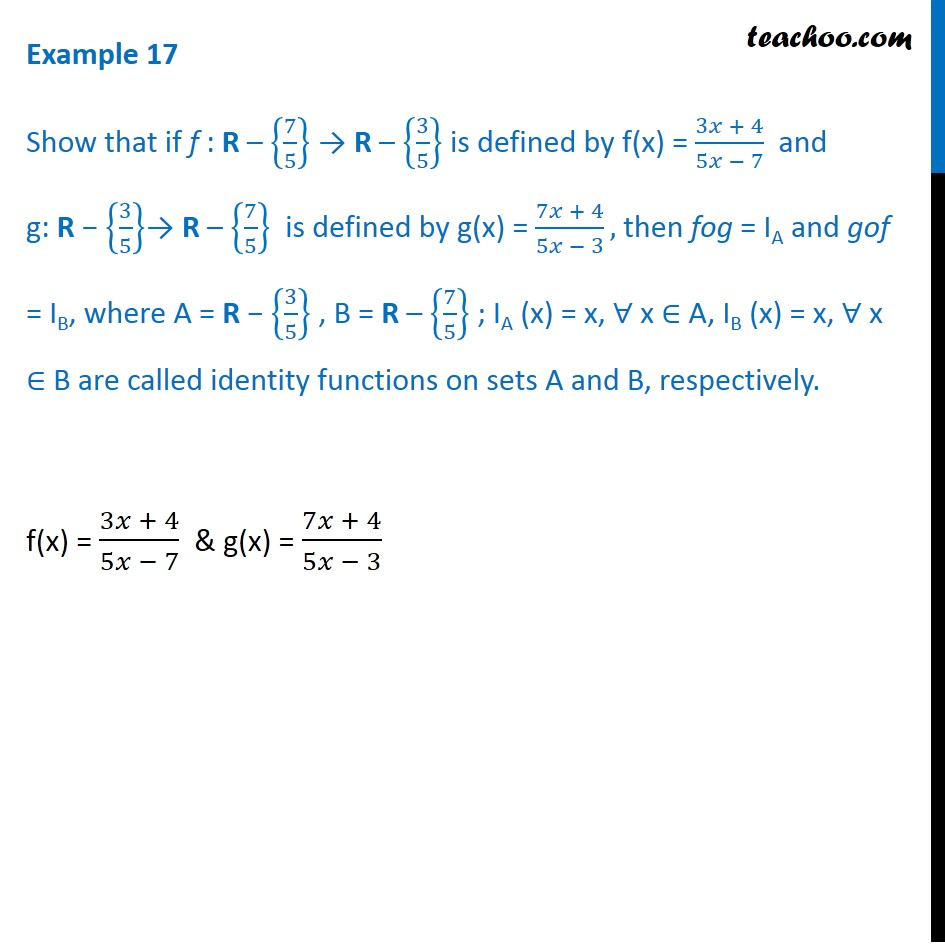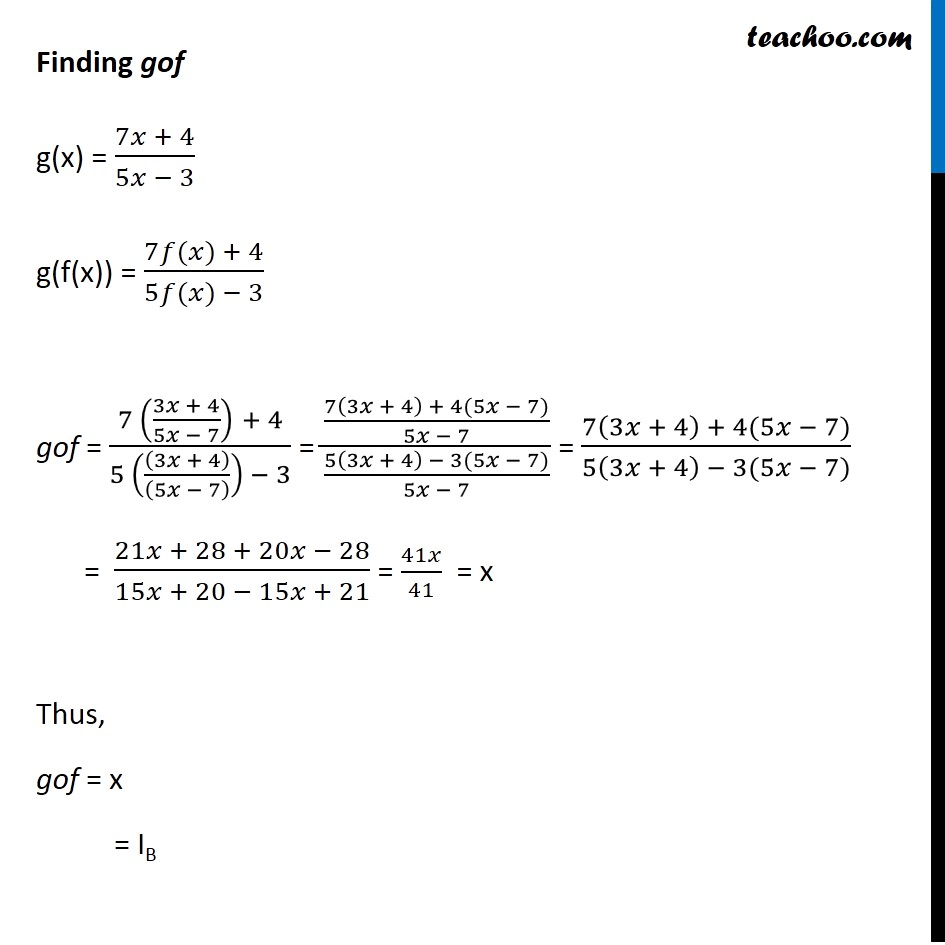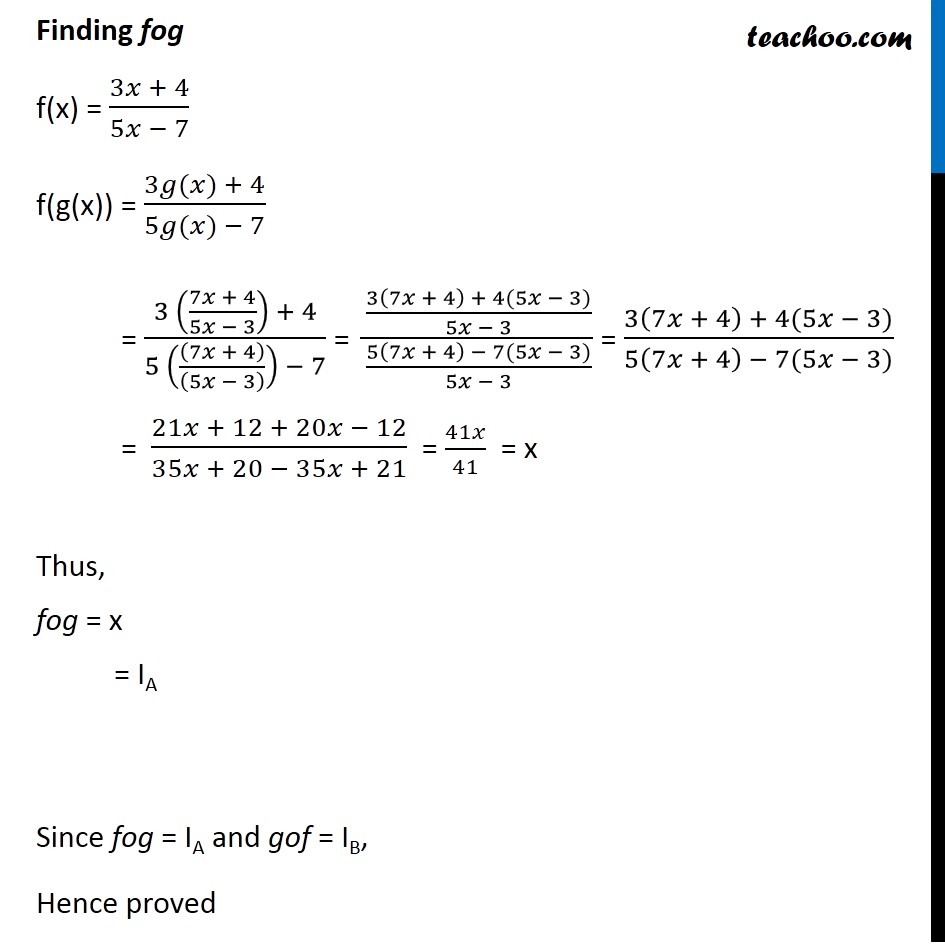Examples

Chapter 1 Class 12 Relation and Functions
Serial order wiseLearn in your speed, with individual attention - Teachoo Maths 1-on-1 Class

### Transcript

Question 1 Show that if f : R – {7/5} → R – {3/5} is defined by f(x) = (3𝑥 + 4)/(5𝑥 − 7) and g: R − {3/5}→ R – {7/5} is defined by g(x) = (7𝑥 + 4)/(5𝑥 − 3), then fog = IA and gof = IB, where A = R − {3/5} , B = R – {7/5} ; IA (x) = x, ∀ x ∈ A, IB (x) = x, ∀ x ∈ B are called identity functions on sets A and B, respectively. f(x) = (3𝑥 + 4)/(5𝑥 − 7) & g(x) = (7𝑥 + 4)/(5𝑥 − 3) Finding gof g(x) = (7𝑥 + 4)/(5𝑥 − 3) g(f(x)) = (7𝑓(𝑥) + 4)/(5𝑓(𝑥) − 3) gof = (7 ((3𝑥 + 4)/(5𝑥 − 7))" " + 4)/(5 (((3𝑥 + 4))/((5𝑥 − 7) )) − 3) = ((7(3𝑥 + 4) + 4(5𝑥 − 7))/(5𝑥 − 7))/((5(3𝑥 + 4) − 3(5𝑥 − 7))/(5𝑥 − 7)) = (7(3𝑥 + 4) + 4(5𝑥 − 7))/(5(3𝑥 + 4) − 3(5𝑥 − 7)) = (21𝑥 + 28 + 20𝑥 − 28)/(15𝑥 + 20 − 15𝑥 + 21) = 41𝑥/41 = x Thus, gof = x = IB Finding fog f(x) = (3𝑥 + 4)/(5𝑥 − 7) f(g(x)) = (3𝑔(𝑥) + 4)/(5𝑔(𝑥) − 7) = (3 ((7𝑥 + 4)/(5𝑥 − 3)) + 4)/(5 (((7𝑥 + 4))/((5𝑥 − 3) )) − 7) = ((3(7𝑥 + 4) + 4(5𝑥 − 3))/(5𝑥 − 3))/((5(7𝑥 + 4) − 7(5𝑥 − 3))/(5𝑥 − 3)) = (3(7𝑥 + 4) + 4(5𝑥 − 3))/(5(7𝑥 + 4) − 7(5𝑥 − 3)) = (21𝑥 + 12 + 20𝑥 − 12)/(35𝑥 + 20 − 35𝑥 + 21) = 41𝑥/41 = x Thus, fog = x = IA Since fog = IA and gof = IB, Hence proved